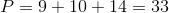# GMAT Math : Calculating the perimeter of an acute / obtuse triangle

## Example Questions

### Example Question #1 : Acute / Obtuse Triangles

A triangle has 2 sides length 5 and 12.  Which of the following could be the perimeter of the triangle?

I. 20

II. 25

III. 30

II and III only.

III only

I only

All 3 are possible.

I and II only

II and III only.

Explanation:

For a triangle, the sum of the two shortest sides must be greater than that of the longest.  We are given two sides as 5 and 12.  Our third side must be greater than 7, since if it were smaller than that we would have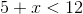whereis the unknown side.  It must also be smaller than 17 since were it larger, we would have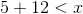.

Thus our perimeter will be between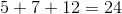and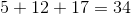. Only II and III are in this range.

### Example Question #2 : Acute / Obtuse Triangles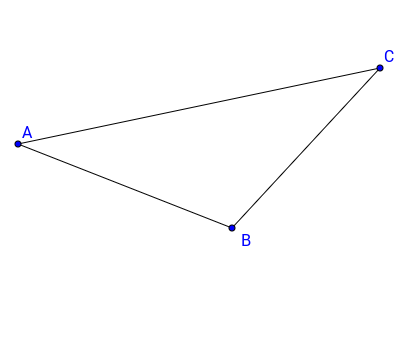Triangle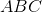has sides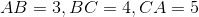. What is the perimeter of triangle?Explanation:

To calculate the perimeter, we simply need to add the three sides of the triangle.

Therefore, the perimeter is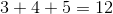, which is the final answer.

### Example Question #3 : Acute / Obtuse Triangles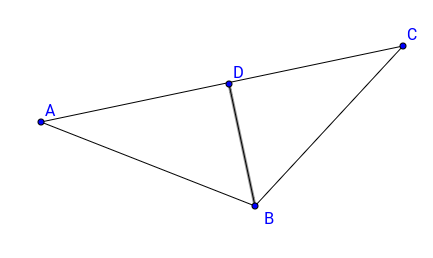Trianglehas height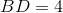. Ifis the midpoint of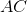and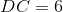, what is the perimeter of triangle?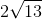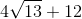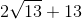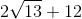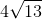Explanation:

Since BD is the height of triangle ABC, we can apply the Pythagorean Theorem to, let's say, triangle DBC and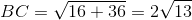.

Since the basis of the height is at the midpoint of AC, it follows that triangle ABC, is an isoceles triangle.

We can find the perimeter by multiplying BC by 2 and add the basis of the triangle AC, which has length of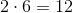.

The final answer is therefore.

### Example Question #4 : Acute / Obtuse Triangles

An acute triangle has side lengths of, and. What is the perimeter of the triangle?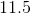Explanation:

For any given triangle, the perimeteris the sum of the lengths of its sides. Given side lengths of, and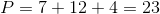### Example Question #5 : Acute / Obtuse Triangles

An acute triangle has side lengths of, and. What is the perimeter of the triangle?Explanation:

For any given triangle, the perimeteris the sum of the lengths of its sides. Given side lengths of, and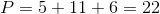### Example Question #6 : Acute / Obtuse Triangles

An acute triangle has side lengths of, and. What is the perimeter of the triangle?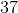For any given triangle, the perimeteris the sum of the lengths of its sides. Given side lengths of, and